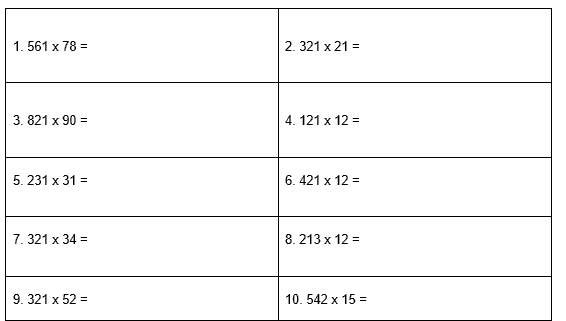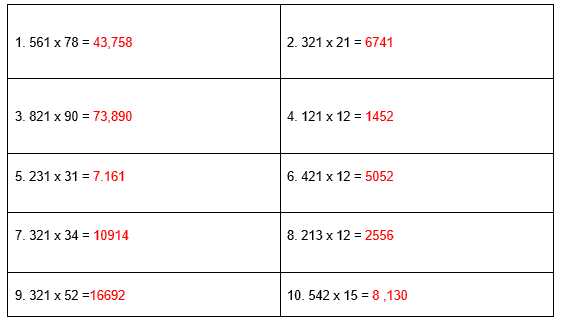# 3-Digit by 2-Digit Multiplication Worksheet (with answer key + PDF)

In mathematics, three-digit multiplication is the process of multiplying three-digit numbers by two-digit, one-digit, or three-digit numbers by arranging the numbers in columns based on their place values. When compared to 2-digit or 1-digit multiplication, three-digit multiplication advances.

## What is the “3-Digit by 2-Digit Multiplication Worksheet”?

This worksheet will explore some of the 3-digit by 2-digit multiplication. A technique for multiplying 3-digit numbers with other numbers is known as 3-digit multiplication. When multiplying three-digit numbers, we place the digits in columns based on their place values.

This worksheet will help the students to comprehend the topic. Also, in this worksheet, we will practice the multiplication of three digits by one digit, three digits by two digits, and three digits by three digits. We will also solve a few examples to help us understand the concept.

## Instructions on how to use the “3-Digit by 2-Digit Multiplication Worksheet.”

Worksheets for 3-digit by 2-digit multiplication help children gain confidence in their ability to multiply larger numbers as well as problem-solving skills. Study the concept and examples given and try to solve the given exercises below.

## Conclusion

Worksheets for 3-digit by 2-digit multiplication help students learn the additional facts and gain an understanding of the multiple-digit multiplication concepts. The commutative property is a crucial concept that children learn as they master the multiplication facts in multiple-digit multiplication worksheets.

If you have any inquiries or feedback, please let us know.

## 3-Digit by 2-Digit Multiplication Worksheet (with answer key + PDF)

3-Digit by 2-Digit Multiplication

We first write the 3-digit number on top, followed by the 2-digit number, in order to multiply 3-digit numbers by 2-digit numbers. In the sections that follow, we’ll go over 3-by-2-digit multiplication both without and with regrouping.

3-Digit by 2-Digit Multiplication Without Regrouping

When we multiply a 3-digit number by a 2-digit number, we first multiply the multiplier’s one’s digit by the multiplicand, followed by the multiplicand’s tens digit. The final product is created by combining both of these products.

Example: Multiply 311 by 21.

Solution: Let us multiply 311 by 21 stepwise.

• Step 1: Arrange the numbers 311 and 21 in columns according to their place values as shown below.
• Step 2: Multiply 1 by each digit of 311.
• When 1 is multiplied by 1, we get 1.
• When 1 is multiplied by 1, we get 1.
• When 1 is multiplied by 3, we get 3. So, we have 311 as the first partial product.
• Step 3: Now, we place a zero under the first partial product, that is, just before we write the second partial product in the next line. This 0 is placed here because in this step we are actually multiplying 311 by 21.
• Step 4: Multiply 3 by each digit of 311.
• When 2 is multiplied by 1, we get 2.
• When 2 is multiplied by 1, we get 2.
• When 2 is multiplied by 3, we get 6. So, we have 6220 as the second partial product.
3 Digit by 2 Digit Multiplication